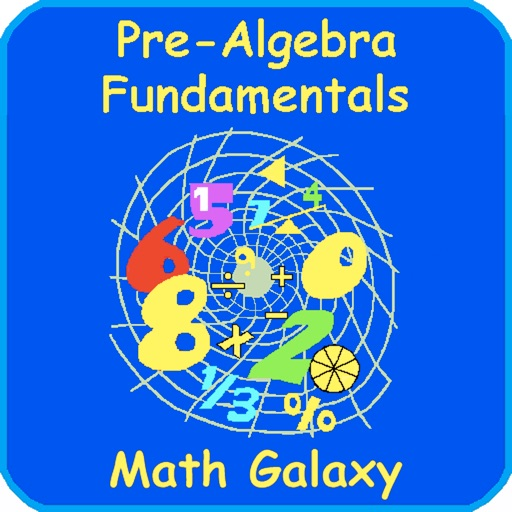Pre-Algebra Fundamentals# Pre-Algebra Fundamentals

\$4.99
0

0 Ratings

Update Date

2019-06-21

Size

91.0 MB

### Screenshots for iPhone

iPhone
Pre-Algebra Fundamentals Description
Math Galaxy Pre-Algebra Fundamentals is like having an interactive textbook, with explanations, practice, games, and videos that cover all topics found in middle school math:

decimals
negative numbers (integers)
absolute value
powers/exponents
scientific and metric notation and operations
order of operations
factors and prime numbers
1 & 2-step equations
slopes and linear equations
ratios & proportions
similar figures
direct & inverse variation
percents
geometry, including length, area, volume, weight, lines, angles & polygons
pythagoras & right triangles
basic trigonometry
graphs
frequency tables,
mean (average) & median
stem-and-leaf plots
box-and-whisker plots
probability
patterns and sequences

The concepts are presented in bite-size pieces and there is an unlimited number of practice problems, with numbers chosen randomly.

By solving problems students earn robots to use in a maze game called the Labyrinth.
Pre-Algebra Fundamentals 2.1 Update
2019-06-21 Version History
Fixed repetition of problems in Ratios & Proportions exercise.
More
Price:
\$4.99
Version:
2.1
Size:
91.0 MB
Update Date:
2019-06-21
Developer:
Math Galaxy
Language:
English
More
You May Also Like
Developer Apps